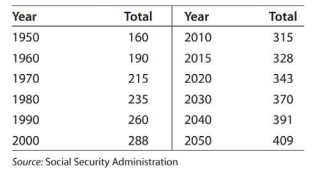# Modeling U.S. population The table gives the U.S. population to the nearest million tor selected years from 1950 and projected to 2050. Find a cubic function P ( t ) that models these data, where P is the U.S. population in millions and t is the number of years past 1950. Report the model with three significant digit coefficients. Use the part (a) result to find the function that models the instantaneous rate of change of the U.S. population. Find and interpret the instantaneous rates of change in 2000 and 2025.### Mathematical Applications for the ...

11th Edition
Ronald J. Harshbarger + 1 other
Publisher: Cengage Learning
ISBN: 9781305108042

#### Solutions

Chapter
Section### Mathematical Applications for the ...

11th Edition
Ronald J. Harshbarger + 1 other
Publisher: Cengage Learning
ISBN: 9781305108042
Chapter 9.4, Problem 61E
Textbook Problem
1 views

## Modeling U.S. population The table gives the U.S. population to the nearest million tor selected years from 1950 and projected to 2050.Find a cubic function P ( t ) that models these data, where P is the U.S. population in millions and t is the number of years past 1950. Report the model with three significant digit coefficients. Use the part (a) result to find the function that models the instantaneous rate of change of the U.S. population. Find and interpret the instantaneous rates of change in 2000 and 2025.

This textbook solution is under construction.

### Still sussing out bartleby?

Check out a sample textbook solution.

See a sample solution

#### The Solution to Your Study Problems

Bartleby provides explanations to thousands of textbook problems written by our experts, many with advanced degrees!

Get Started